# 1st PUC Chemistry Question Bank Chapter 7 Equilibrium

## Karnataka 1st PUC Chemistry Question Bank Chapter 7 Equilibrium

### 1st PUC Chemistry Equilibrium One Mark Questions and Answers

Question 1.
Mention factors affecting rate (velocity) of reaction.

1. Nature of reactants
2. Catalyst
3. Temperature
4. Pressure
5. Concentration of reactants.

Question 2.
Define Law of mass action.
“At a given temperature the rate of chemical reaction is directly proportional to the product of active masses of the reactant.”

Question 3.
Define equilibrium constant of reaction.
“Is the ratio of velocity constant of forward reaction to velocity constant of backward reaction.” Also defined as, “It is the ratio of product to molar concentration of product to the product of molar concentration of the reactants”.Question 4.
Write expression for Kc & KP for the reaction.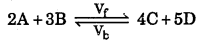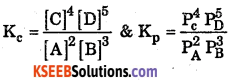Question 5.
Write the relationship between Kp & Kc.
Kp = Kc [RT] Δn
Where Δn is = (number of gaseous product – number of gaseous reactant)

Question 6.
Define Le-Chatelier’s principle.
Definition : “when a constraint applied to a system at equilibrium in a reversible reaction, the equilibrium shifts so as to nullify the constraint”.
[Constraint is change in temperature or pressure, addition of reactant or product]

Question 7.
What is active mass ?
Active mass means molar concentration i.e., concentration expressed in mol/dm3.

Question 8.
What happens when the temperature of a reversible reaction at equilibrium is increased, if enthalpy change is positive?
Equilibrium shifts to the right.Question 9.
Give an example for a reversible reaction in which Kp = KcRT.
PCI5(g) ⇌ PCI3(g)+CI2(g)

Question 10.
Write an expression for Kp for the following reaction: A + B ⇌ C + 2D.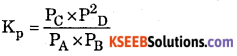Question 11.
How are KP and Kc related ? Mention the condition under which KP=KC.
Kp = Kc (RT)Δn when Δn = 0 i.e. no. of moles of gaseous products = no. of moles of
gaseous reactants, e.g., H2(g) + I2(g) ⇌ 2HI(g)Kp = Kc

Question 12.
Give an example of a reaction where Kp ≠ Kc.
PCI5(g) ⇌ PCI3(g) + CI2(g)

Question 13.
How does a catalyst influence the equilibrium constant of a reversible ‘ reaction ?
A catalyst does not influence the equilibrium constant of reversible reaction.

Question 14.
Which measurable property becomes constant in water , ~~ water vapour
equilibrium at constant temperature?
Vapour pressure.

Question 15.
Give one example of everyday life in which there is gas y..’ >. solution
equilibrium.
Soda-water bottle.

Question 16.
Under what condition, reversible process becomes irreversible?
If one of the products (gaseous) is allowed to escape out (i.e., in open vessel).

Question 17.
What is the effect on the value of equilibrium constant on adding catalyst?
No effect.Question 18.
What is the effect of increasing pressure on the equilibrium?
N2 + 3H2 ⇌ 2NH3 ?
Equilibrium will shift in the forward direction forming more of ammonia.

Question 19.
What are the conditions for getting maximum yield of NHs by Haber’s process?
High concentrations of N2 and H2, low temperature, high pressure.

Question 20.
What happens to the dissociation of PCI5 in a closed vessel if helium gas is introduced into it at the same temperature?
No effect.

Question 21.
What is the law called which gives relationship between degree of dissociation of a weak electrolyte and its concentration in the solution?
Ostwald’s dilution law.

Question 22.
Write the expression for comparison of relative strengths of two weak acids in terms of their ionization constants.Question 23.
What do you mean by reversible process?
Reversible process is a process in which products can react to form reactants back.

Question 24.
Define equilibrium.
Equilibrium is a stage at which rate of forward reaction is equal to rate of backward reaction.

Question 25.
What is physical equilibrium? Give one example.
Physical equilibrium is am equilibrium between two different physical states of same
substance, e.g., H2O(s) ⇌ H2O(I)

Buffer capacity can be also defined as quantity of strong acid or base that must be added to change the pH of one liter of solution by one pH unit.

Question 26.
Give two examples for Acedic buffer.
A solution containing a weak acid and its salt with a strong base.
(i) CH3COOH + CH3COONa
(ii) HCOOH + HCOOKQuestion 27.
Write expression of Kc for reaction N2 (g) + 3H2 (g) ⇌ 2NH3 (g). Give units of K.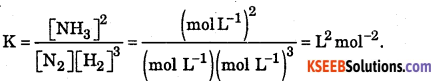Question 28.
Name the factors that affect equilibrium position of a reversible reaction.
(i) Temperature (ii) Pressure, (iii) Concentration, (iv) Catalyst.

Question 29.
At what temperature the solid, and liquid are in equilibrium under 1 atm. pressure?
Melting point or freezing point.

Question 30.
Define solubility of substance.
Solubility of substance is maximum amount of solute that can be dissolved lOOg of solvent at a given temperature.

Question 31.
State Henry’s law.
Henry’s law states the solubility of gas is directly proportional to the partial pressure of the gas above the solution, i.e, mass of gas dissolved is directly proportional to pressure applied on gas.
m = k x p where ‘p’ is pressure applied on the gas.

Question 32.
What is the effect of temperature on solubility of gases in liquids?
Solubility of gases in liquids decreases with increase in temperature.

Question 33.
What is the effect of increasing pressure in the reactions?
(i) PCI5 (g) ⇌ PCI3 (g) + CI2 (g)
(ii) N2 (g)+ O2(g) ⇌ 2NO(g).
(i) The equilibrium will shift in backward reaction.
(ii) No effect.Question 34.
What is the effect of temperature on the reactions?
(i) N2(g) + 3H2(g) ⇌ 2NH3(g) + Heat
(ii) N2(g) + O2(g) ⇌ 2NO3(g) – Heat
(i) The increase in temperature will favour backward reaction because the reaction is exothermic.
(ii) The increase in temperature favours forward reaction because the reaction is endothermic.

Question 35.
Write expression of KP for the reaction.
N2O(g) ⇌ 2NO2(g)Question 36.
N2 (g) + 3H2 (g) ⇌ 2NH3 (g),K = 0.50 at 673K.
Write the equilibrium expression and equilibrium constant for reverse reaction.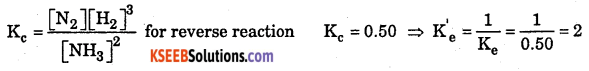Question 37.
What is difference between strong electrolyte, and weak electrolyte?
Strong electrolytes can dissociate into ions completely in aqueous solution whereas weak electrolytes do not dissociate into ions completely and there exist an equilibrium between ioins and unionized molecules, called Ionic Equilibrium.

Question 38.
Define degree of ionization or dissociation.
It is the ratio of number of ions or molecules produced to the total number of molecules.

Question 39.
State Ostwald’s dilution law.
Ostwald’s dilution law states that the degree of dissociation of weak electrolyte is inversely proportional to square root of its concentration.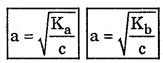Where Ka is acid dissociation constant and Kb is base dissociation constant.Question 40.
Define ionic equilibrium.
The equilibrium between ions and unionized molecules is called ionic equilibrium.

Question 41.
Under what conditions a weak electrolyte can »have high degree of ionization?
On dilution, i.e., at a very low concentration, weak electrolyte has high degree of ionization.

Question 42.
What is the effect of temperature on degree of dissociation?
The degree of Dissociation increases with increase in temperature.

Question 43.
Define pH. Give its mathematical expression.
pH is defined as negative logarithm of [H2O+]concentration.

Question 44.
What is meant by ionic product of water (Ka)?
It is product of concentration of [H3O+] and [OH] at a specific temperature.
Kw = [H3O+ ][OH] = 1.0 × 10-14 at 298K

Question 45.
In qualitative analysis, on what basis cations are grouped?
Cations are grouped on the basis of their solubility product (Ksp).Question 46.
What is the range of a pH indicator in terms of its dissociation constant (Kin)?
pH = pKin ±1.

Question 47.
What happens to the solubility of Agcl in water if NacI solution is added to it?
Solubility of AgCI decreases due to common ion effect.

Question 48.
What is the realationship between pKa and pKb values where Ka and Kb represent ionization constants of the acid and its conjugate base respectively?
pKa + pKb = pKw = 14.

Question 49.
What is the relationship between pH and pOH?
pH + pOH = pKw = 14.

Question 50.
Write the demerits of Brownsted-Lowry theory.
It does not explained why CaO, NH3 acts as base and BF3 and CO2 acts as acid.

Question 51.
Define acidolysis.
Acidolysis is the process where acidity of blood decreases from 7.3.

Question 52.
Define Alkolysis.
Alkolysis is the process where acidity of blood increases from 8.Question 53.
What do you mean by buffer solution?
The solution which resist the pH or pOH when an acid or base is added to it. Buffer solution is a mixture of weak electrolyte and its salt.

Question 54.
Define buffer action.
The ability or capacity of a buffer solution to resist the pH by addition of acid or base.

Question 55.
Mention the types of buffer solution.
acidic buffer
basic buffer
neutral buffer.

Question 56.
Mention the uses of buffer solution.
It is used in chemical industries like paper, sugar, pharmaceuticals.
It is used in all biological fluids to maintain constant pH by buffer action.### 1st PUC Chemistry Equilibrium Two Marks Questions and Answers

Question 1.
Explain rate of reaction with its mathematical forms.
Rate (Velocity) of reaction:
It is the ratio of change in concentration to unit interval of time.
Mathematically: Let us consider a general reaction, A (reactant) → B(products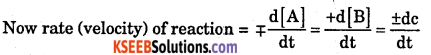Where dc = change in concentration : dt = change in time.
-ve sign = forward reaction where concentration of reactant decreases.
+ve sign = backward reaction where concentration of reactant increases.
Meaning of [A] = – concentration of reaction A
[B] = – concentration of Product B.

Question 2.
Define Rate equation with example.
An expression which relates the rate (velocity) of the reaction with change in concentration of reactant in a given interval of time.Question 3.
Define Irreversible reaction with example.
A Chemical reaction in which product can not able to give back the reactant are called Irreversible reaction.
Example : C(s) + O2 (g) → CO2 (g)

Question 4.
Define Reversible reaction with example.
A Chemical reaction in which an infinitesimally small change in any one of the variable (Pressure, Temperature, Concentration of reactant or product) of the reaction is changed the direction (forward or backward) of the reaction changes.”
It is repreented by half arrow mark. An Example is H2 (g) +I2 (g) ⇌ 2HI(g)Question 5.
Define Chemical Equilibrium with an example.
Is a state where the rate of forward reaction is equal to rate of backward reaction.” OR is also defined as “ A state where concentration of reactant and product remains
constant. ” Example : H2 (g) +I2 (g) ⇌ HI(g)

Question 6.
Chemical equilibrium is dyanamic. Justify.
Chemical equilibrium is dynamic : Because the velocities of forward and backward reactions are equal also the concentration of reactant and product unchanged at this equilibrium state, (but may not be equal). The equilibrium can be made to shift in either direction by changing the concentration. Pressure, Volume etc., Hence is dynamic in nature.

Question 7.
Write the characteristics of chemical equilibrium.

• Chemical equilibrium is dynamic.
• Equilibrium reaction will not come to an end.
• Equilibrium is attained only in a closed system.
• Catalyst does not alter equilibrium state.

Question 8.
The equilibrium constant for a reaction is 26.66. If the rate constant of the forward reaction is 3.2 × 10-4 s-1, what is the rate constant of the backward reaction?
Data given,
Equilibrium constant for a reaction Kc – 26.66
Rate constantof the forward reaction = k1= 3.2 × 10-4 /sec.
∴ Rate constant of the backward reaction = k2 = ?
Equilibrium constant of a reaction ‘Kc’ is given by the formula.Question 9.
Define Arrhenius acid – base theory with one example.
An electrolyte which when dissolved in water, produces H+ ion is called acid.
An electrolyte which when dissolved in water, produces OH ion is called base.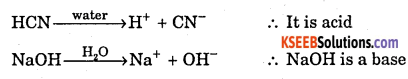Question 10.
Define Bronsted Lowry theory OR Protonic theory with one example.
A substance or molecule which donate proton is called acid [Protogenic]
A substance or molecule which accepts proton is called base [Protophillic]
Example: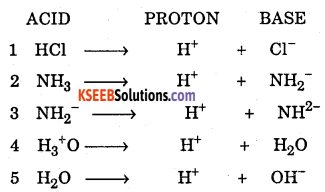Question 11.
What are amphoteric substance? Give one example.
A substance or molecule which acts both as acid as well as base are called amphoteric.
Example:Question 12.
What are conjugate acid-base pair? Give one example.
Acid – base pairs which differed by one proton is called conjugate acid – base pair.
NH3+H2O → NH4+ + OH
Conjugate base of acid H2O is OH ; H2O + H2O → H3O+ + OH
Conjugate acid of base NH3 is NH3+ ; NH3 +NH3 → NH4+ +NH2.

Question 13.
Explain Lewi’s electron acid – base concept with an example.
According to Lewi’s theory, acid is one which accepts pair of electron and base is a – substance which donates pair of electron.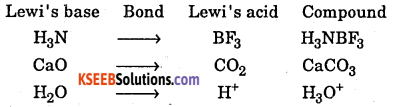Question 14.
Calculate pH of 0.01 M HC1.
pH = -log10[H+] = -log10[0.01] = -log10[10-2] = 2

Question 15.
Calculate pH of 0.0001 M of HNO3.
pH = -log10[H+ ]-log10[0.0001] = -log10[10-4] = 4

Question 16.
Calculate pH of 0.00025 M HNO3.
pH = -log10[H+] = -Iog10[0.00025] – log10 [2.5 × 10-4] = 4 – log2.5
pH =4 – 0.3979 = 3.6021Question 17.
Calculate pH of 0.1 M of H2SO4 (concentration 0.1 × 2 hydrogen = 0.2).
pH = -log10[H+] = -log10[0.1 × 2] = -log10[0.2] = -log10[2 × 10-1] = 1 – log2
pH = 1 – 0.3010 = 0.699

Question 18.
Calculate pH of 0.05 M HC1.
pH = -log10[H+] = -log10[0.05] – log10 [5 × 10-2] = 2 – log5 = 2 – 0.6990 =1.301

Question 19.
Calculate pH of 0.005 M H2SO4.
pH = -log10[H+] = -log10[0.005 × 2] = -log10[0.01] = -log10[ 1 × 10-2 ]
pH = 2 – log 1 = 2 – 0 = 2

Question 20.
Calculate pH of 3 × 10-9 M NaOH.
pOH = -log10[OH] = -log10[3 × 10-9] = 9 – log3 = 9 – 0.4771 = 8.5229

Question 21.
Calculate [OH] if pOH = 8.3.
pOH =-log10[OH]; 8.3 =-log10[OH]
Taking antilog on both the sides
[OH] = antilog (-8.3) = [OH] = antilog (-9 – 8.3 + 9) = antilog (0.7) × 10-9]
= 5.012 × 10-9 mol/dm3

Question 22.
Calculate [H+] if pOH = 9.23.
pH + pOH = 14 pH = 14 – pOH = 14 – 9.23 = 4.77
pH = -log10[H+] = -4.77 = log[H+]
Taking antilog on both the sides
[H+] = antilog (-4.77) = antilog [+5 -4.77 – 5] = antilog [0.23] × 10-5
= 1.698 × 10-5] mol/dm3]

Question 23.
Calculate [OH] if pH = 5.284
pH = -log10[H+]; [H+] = antilog [-5.284] = antilog [6 – 5.284 – 6]
= antilog (0.716 – 6) = 5.2 × 10-6 mol/dm3Question 24.
What is the pH of a 0.05 M solution of formic acid ? (Ka = 1.8 × 10-4)Question 25.
Calculate the [OH ] of a solution whose pH is 9.62.
pH + pOH = 14 pOH = 14 – pH = 14 – 9.62 = 4.38
pOH = 4.38 = -log10[OH]; 4.38 = -log10[OH”]
log10[OH] = -4.38; [OH-] = antilog (-4.38) = antilog (5 – 4.38 – 5)
= antilog (0.62 – 5) = antilog (0.62) × 10-5 = 4.169 × 10-5 mol dm-3.

Question 26.
The pOH of a solution is 5.725. Calculate the [H+].
pH + pOH = 14 pH = 14 – pOH = 14 – 5.725 = 8.275
pH = -log10[H+] ⇒ 8.275 = log10[H+] ⇒
[H+] = antilog (-8.275) = antilog(9 – 8.275 – 9).
[H+] = antilog (0.275 – 9) = 5.309 × 10-9 mol dm-3.

Question 27.
Calculate the pH of 0.125M H2SO4.
H2SO4 → 2H+ +SO42-
[H+] = 2[H2SO4] = 2 × 0.125 = 0.25
pH = -log10[H+] = -log10[0.25] = -log10 [2.5 × 10-1]
pH =-log2.5-log10-1 =-0.3979+1 = 0.6021.

Question 28.
Solubility product of barium sulphide (BaS04) is 2.4 × 10-9. Calculate it’s solubility.
BaSO4 is AB type of salt
Ksp = 2.4 × 10-9Question 29.
If the solubility product of silver chloride is 1.8 × 10-10. What is the solubility of siliver ion if concentration of Cl is 0.01 molar.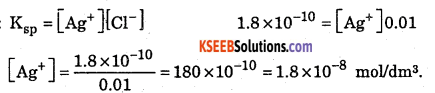Question 30.
Give any two differences between strong and weak electrolyte.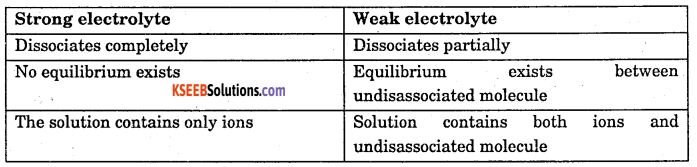Question 31.
What is common ion effect ? Give example.
Supression in degree of dissociation of a weak electrolyte by addition of a common ion is called common ion effect.
Example : CH3COOH and CH3COONa### 1st PUC Chemistry Equilibrium Three Marks Questions and Answers

Question 1.
Some processes are given below. What happens to the process if it subjected
to a change given in the brackets?
(i)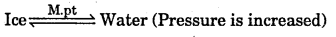(ii) Dissolution of NaOH in water (Temperature is increased)
(iii) N2 (g) + O2 (g) ⇌ 2NO(g) -180.7kJ (Pressure is increased and temperature is decreased)
(i) Equilibrium will shift in the forward direction, i.e., more of ice will melt.
(ii) Solubility will decrease because it is an exothermic process.
(iii) Pressure has no effect. Decrease of temperature will shift the equilibrium in the backward direction.Question 2.
What qualitative information can you obtain from the magnitude of equilibrium constant?
(i) Large value of equilibrium constant (>103) shows that forward reaction is favoured, i. e., concentration of products is much larger than that of the reactants at equilibrium.
(ii) Intermediate value of K(10-3 to 103) shows that the concentration of the reactants and products are comparable,
(iii) Low value of K(<10-3)shows that backward reaction- is favoured, i.e, concentration of reactants is much larger than that of the products.

Question 3.
How do you explain detection of I group basic radicals based on application and solubility product.
Group reagent: Dil. HCl → H+ + Cl
Redicals present: Pb2+, Hg2+, Cd2+
The group reagent HCl is a strong electrolyte under goes complete dissociation. The metal radicals forms it’s respective chlorides [PbCl2, HgCl2, CdCl2 ] Whose solubility product is less than ionic product. Hence, forms pricipitate.

Question 4.
How do you explain detection of H group basic radicals based on application of solubility product.
Group reagent: HCl → H+ + Cl; H2S ⇌ 2H+ + S2-

Here HCl is a strong electrolyte under goes complete dissociation where as H2S is a weak electrolyte under goes partial dissociation and attains equilibrium between it’s ions and molecules.

In this solution, because of common ion (H+) effect supression of degree of dissociation of H2S takes place. As a result [S2-] decreases. Hence the metal radical forms it’s respective sulphides [CuS, SnS, BiS] whose solubility product is less than ionic product. Hence forms pricipitate.Question 5.
How do you explain the detection of HI group basic radicals based on application of solubility product ?
Group reagent: NH4OH ⇌ NH4+ + OH ; NH4Cl ⇌ NH4+ + Cl
Here NH4Cl is a strong electrolyte under goes complete dissociation where as NH4OH is a weak electrolyte under goes partial dissociation and attains equillibrium between it’s ions and molecules.

In this solution because of common ion (NH4+) effect supression of degree of dissociation of NH4OH takes place. As a result [OH] decreases. Hence the metal radical forms it’s respective hydroxides [Al(OH)3, Cr(OH)3, Fe(OH)3] Whose solubility product is less than ionic product. Hence forms precipitate.

Question 6.
Explain detection of IV group basic radicals using application of solubility product.
Group reagent: NH4Cl → NH4+ + Cl ; NH4OH ⇌ NH4+ + OH
H2S ⇌ 2H+ + S2- ; H+ + OH → H2O

Here, NH4Cl is a strong electrolyte undergoes complete dissociation, where as NH4OH – and H2S are weak electrolytes undergoes partial dissociation and attains equillibrium between their ions and molecules.

Along with common ion (NH4+) effect, the H+ and OH ion combines to form water molecules.
At the same time the metals forms their respective sulphides [ZnS, MnS] Whose solubility product is less than ionic product. Hence, forms precipitate.

Question 7.
Define Arrhenius acid – base theory with one example.
An electrolyte which when dissolved in water, produces H+ ion is called acid.
An electrolyte which when dissolved in water, produces OH- ion is called base.Question 8.
Deduce equation for Ostwalds dilution law with respect to the weak acid.
Consider a weak acid HA with concentration C mol/dm3. After a time interval it reaches equillibrium with dissociation x mol/dm3 as follows.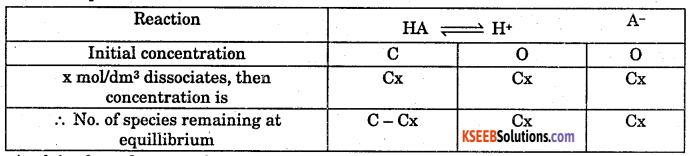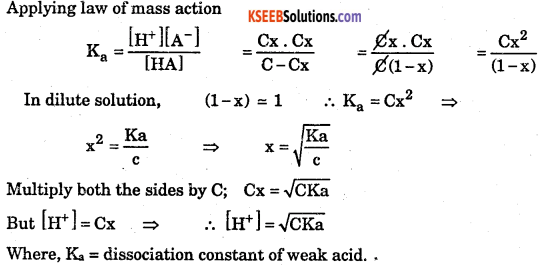Question 9.
Deduce equation for Ostwalds dilutions law with respect to weak base.
Consider a weak base BOH with concentration C mol/dm3. After a time internal it reaches equilibrium with dissociation x mol/dm3 as follows.(ii) Where, Kb is dissociation constant of weak base.

Question 10.
Derive ionic product of water. Also find the value at 25 °C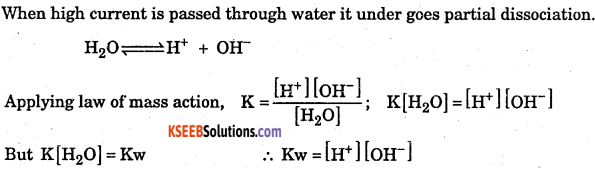Where, Kw is ionic product of water.
Value of Kw at 25°C :
It is found that at 25°C [H+] = [OH] = 10-7 mol/dm3
∴Kw = [H+][OH] = 10-7 × 10-7 = 10-14 (mol/dm3)2
(iii) At 25°C the value of Kw is 10-14( tmol/dm3)2### 1st PUC Chemistry Equilibrium Five Marks Questions and Answers

Question 1.
How do you apply law of mass action to a gaseous reversible reaction.
If the reactants and products are in gaseous state, then for the reaction,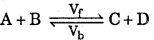The total pressure P = PA + PB + PC + PD
If PA PB PC & PD are represents the partial pressure exerted by A, B, C and D respectively, then according to law of mass action,
Velocity of forward reaction Vf ∝ proportional PA PB Or
Vf=Kf PAPB………….(3)
Where Kf velocity (proportionality) constant of forward reaction.
Velocity of backward reaction Vb ∝ PC PD Or
Vb = Kb PCPD ……….(4)
Where Kb velocity (proportionality) constant of backward reaction.
At equilibrium, velocity of forward reaction is equal to velocity of backward reaction, from (3) & (4).
Vf = Vb ThenWhere KP is equilibrium constant with respect topartial pressure of the reactant.

Question 2.
Write the characteristics of Equilibrium Constant (KP & KC).

• It is independent of initial concentrations of the reactants, the direction from (reactant or product) which equilibrium attained.
• The value of equilibrium constant independent of Volume, Pressure, and presence of catalyst or any inert material.
• Equilibrium constant gives relative idea about the extent to whick the reaction takes place.
• At a given temperature, the equilibrium constant of a particular reaction has definite value.
• If its (Kc or Kp) value is large, then the forward reaction takes place nearly to completion whereas backward reaction to a small extent Vice versa.
• If the forward reaction is exothermic, then the value of Kc or KP decreases. Vice versa.
• In a reversible reaction, the value of equilibrium constant of forward reaction is reciprocal of backward reaction, ieKf=1/Kb.
• The value of equilibrium constant depends on the stoichiometry of reactants, and products at equilibrium.Question 3.
Write the conjugate acid for the following (a) Cl, (b) NO3, (c) HSO4, (d) HCO3, (e) SO42-, (f) CO32-, (g) NH2-, (h) N3-, (i) O2-, (j) H2O, (k) NH3.Question 4.
Deduce Handersen equation for basic buffer.
Consider the basic buffer, a mixture of ammonium hydroxide and ammonium Chloride.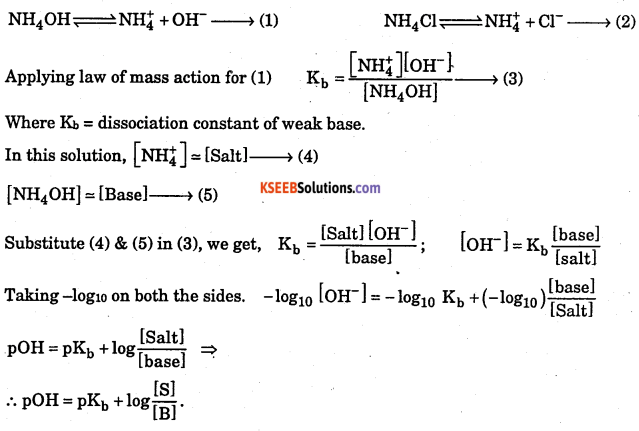Question 5.
Deduce Hendersons equation for acidic buffer.
Consider the acidic buffer, a mixture of acetic acid and sodium acetate as.
CH3COOH ⇌ CH3COO + H+ → (1)
CH3COONa → CH3COO + Na+ →(2)Where, Ka is dissociation constant of weak acid.
In this solution, [ CH3COO ] ≅ [Salt] → (4)
[CH3COOH] ≅ [Acid] → (5)Question 6.
Explain Mechanism or working of acid buffer:
Consider acidic buffer mixture,
CH3COOH ⇌ CH3COO + H+ ; CH3COONa → CH3COO + Na+
Here, acetic acid is a weak electrolyte, in it’s solution there exists equillibrium between it’s ions and molecules. Where as sodium acetate completely dissociates into it’s ions.

Therefore, the buffer mixture contains large number of CH3COO ions followed by Na+, H+, CH3COOH.

Case i) When an acid added to this solution :
H+ ion of the acid combines with CH3COO ion in buffer solution and makes equillibrium with acetic acid.
CH3COO + H+ ⇌ CH3COOH
As a result pH remains constant.

Case ii) When a base is added to this solution :
OH ion of the base combines with H+ ion in buffer solution forms water molecule.
H++ OH → H2O.
As a result pH remains constant.

Question 7.
Explain the Mechanism or working of basic buffer.
Consider basic buffer solution,
NH4OH ⇌ NH4+ + OH ; NH4Cl → NH4 + Cl
Here, ammonium hydroxide is a weak electrolyte, in it’s solution there exist equillibrium between it’s ions and molecules.
Where ammonium chloride completely dissociates into it’s ions.
Therefore, buffer solution contains large number of NH4+ions followed by Cl, OH and NH4OH.

Case (i) When a base is added to this solution:
OH- ion of the base combines with NH4+ ion of the buffer solution and makes equillibrium with ammonium hydroxide.
NH4+ + OH⇌ NH4OH; As a result pOH remains constant.Case (ii) When an acid is added to this solution :
H+ ion of acid combines with OH ion of buffer solution and forms water molecule. H+ + OH → H2O
As a result pOH remains constant.

Question 8.
The pKa value of acetic acid is 4.7447 at 25 °C. How would you obtain a buffer of acetic acid and sodium acetate with pH = 4?So by mixing sodium acetate and acetic acid in the ratio 0.18 : 1 mole per dm3, the buffer of pH = 4 is obtained.

Question 9.
A buffer solution of pH = 4.7 is prepared from CH3COONa and CH3COOH. Dissociation constant of acetic acid is 1.75 × 10-5. Calculate the mole proportion of sodium acetate and acetic acid.Question 10.
A buffer solution of pH 8.3 is prepared from nmnionium chloride and ammonium hydroxide. Dissociation constant of ammonium hydroxide is 1.8 × 10-5. What Is the mole proportion of ammonium chloride and ammonium hydroxide?Question 11.
3.0 g of pure acetic acid and 4.1 g of anhydrous sodium acetate are dissolved together in water and the solution is made up to 500 ml. Calculate the pH of the solution. Given Ka of acetic acid is 1.75 × 10-5.[CH3COOH] $$\frac{3.0 \times 2}{60}=\frac{6.0}{60}$$ = 0.1N